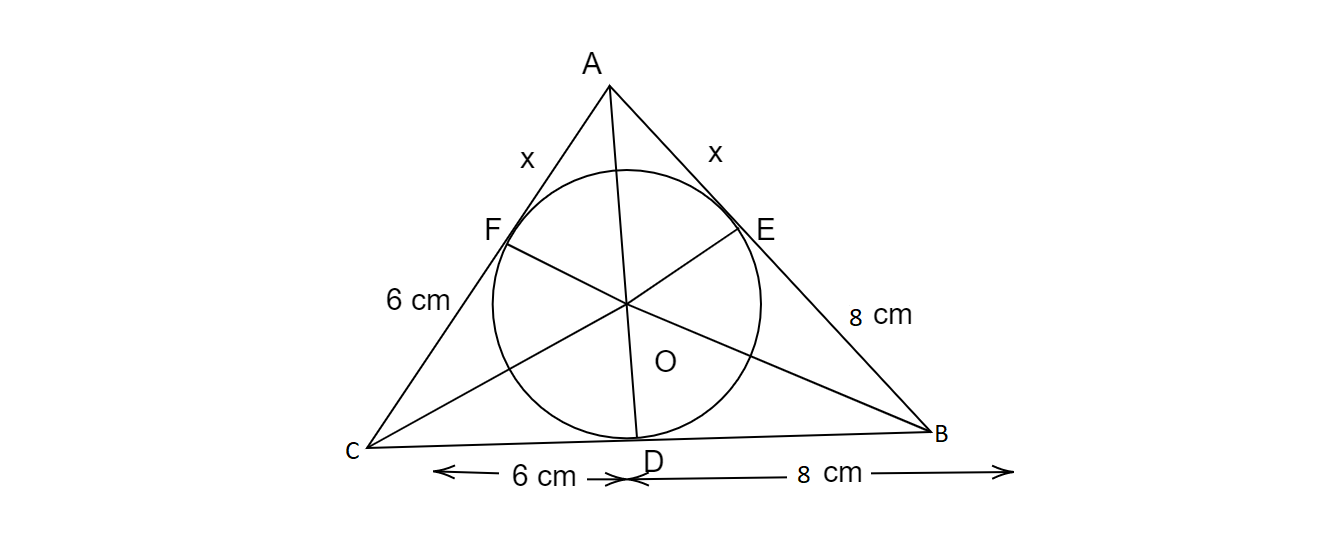" ">

# A triangle $ABC$ is drawn to circumscribe a circle of radius 4 cm such that the segments $BD$ and $DC$ into which $BC$ is divided by the point of contact $D$ are of lengths 8 cm and 6 cm respectively (see figure). Find the sides $AB$ and $AC$."

Given:

A triangle $ABC$ is drawn to circumscribe a circle of radius 4 cm such that the segments $BD$ and $DC$ into which $BC$ is divided by the point of contact $D$ are of lengths 8 cm and 6 cm respectively

To do:

We have to find the lengths of sides $AB$ and $AC$.

Solution:Let the given circle touch the sides AB and AC of the triangle at points $E$ and $F$ respectively and let the

length of line segment $AF$ be $x$.

$AE = AF = x\ cm$
In $∆ABC$,

$a = 6 + 8$

$= 14\ cm$

$b = (x + 6)\ cm$

$c = (x + 8)\ cm$

Therefore,

$s=\frac{a+b+c}{2}$

$=\frac{14+x+6+x+8}{2}$

$=\frac{2 x+28}{2}$

$=(x+14) \mathrm{cm}$

$\operatorname{ar}(\Delta \mathrm{ABC})=\sqrt{s(s-a)(s-b)(s-c)}$

$=\sqrt{(x+14) \times x \times 8 \times 6}$

$=\sqrt{48 x \times(x+14)} \mathrm{cm}^{2}$.........(i)

$\operatorname{ar}(\Delta \mathrm{ABC})=\operatorname{ar}(\Delta \mathrm{OBC})+\operatorname{ar}(\Delta \mathrm{OCA})+\operatorname{ar}(\Delta \mathrm{OAB})$

$=\frac{1}{2} \times 4 \times a+\frac{1}{2} \times 4 \times b+\frac{1}{2} \times 4 \times c$

$=2 a+2 b+2 c$

$=2(a+b+c)$

$=2 \times 2(x+14)$............(ii)

From (i) and (ii), we get,

$\sqrt{48 x(x+14)}=4(x+14)$

$48 x(x+14)=4^{2}(x+14)^{2}$

$48 x(x+14) =16(x+14)^{2}$

$3 x(x+14)=(x+14)^{2}$

$3 x =x+14$

$2 x=14$

$x=7$

$\mathrm{AB}=x+8$

$=7+8$

$=15 \mathrm{~cm}$

$\mathrm{AC} =x+6$

$=7+6$

$=13 \mathrm{~cm}$

Updated on: 10-Oct-2022

51 Views